Next: Damped solution Up: HOW TO DIVIDE NOISY Previous: HOW TO DIVIDE NOISY

## Dividing by zero smoothly

Think of any real numbers x, y, and f and any program containing x=y/f. How can we change the program so that it never divides by zero? A popular answer is to change x=y/f to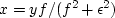, whereis any tiny value. When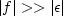,then x is approximately y/f as expected. But when the divisor f vanishes, the result is safely zero instead of infinity. The transition is smooth, but some criterion is needed to choose the value of.This method may not be the only way or the best way to cope with zero division, but it is a good way, and it permeates the subject of signal analysis.

To apply this method in the Fourier domain, suppose X, Y, and F are complex numbers. What do we do then with X=Y/F? We multiply the top and bottom by the complex conjugate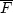,and again add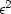to the denominator. Thus,(12)
Now the denominator must always be a positive number greater than zero, so division is always safe.

In preparing figures with equation (12), I learned that it is helpful to recast the equation in a scaled form. First replace,which has physical units of |F|2, by, whereis a dimensionless parameter and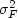is the average value of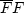.Then I rescaled equation (12) to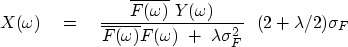(13)
The result is that the scale of X is independent of the scale of F and the scale of.This facilitates plotting X over a range of those parameters. I found the 2s in the expression by experimentation. Of course, if the plotting software you are using adjusts a scale factor to fill a defined area, then the scaling may be unimportant. Equation (13) ranges continuously from inverse filtering with

X=Y/F to filtering with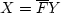,which is called matched filtering.''

Notice that for any complex number F, the phase of 1/F equals the phase of,so all these filters have the same phase.

The filteris called the matched filter." If nature created Y by random bursts of energy into F, then building X from Y and F by choosing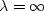in equation (13) amounts to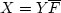which crosscorrelates F with the randomly placed copies of F that are in Y.Next: Damped solution Up: HOW TO DIVIDE NOISY Previous: HOW TO DIVIDE NOISY
Stanford Exploration Project
10/21/1998